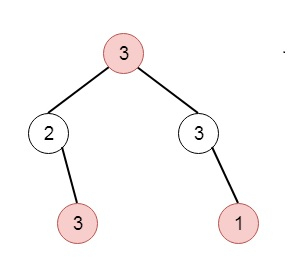# House Robber III in C++

Suppose one thief has found himself a new place for his thievery again. There is only one entrance to this area, the entrance is called the "root." Besides the root, each house has one and only one parent house. After a tour, the smart thief felt that "all houses in this place forms a binary tree". And It will automatically contact the police if two directly-linked houses were broken into on the same night. We have to find the maximum amount of money the thief can rob tonight without alerting the police. So if the tree is like −Then the output will be 7.

To solve this, we will follow these steps −

• Define a method called solve(), this will take the node

• if node is null, then return a pair (-infinity, 0)

• leftVal := left of node, rightVal := right of node

• first element of leftVal := maximum of first element of leftVal and 0

• second element of leftVal := maximum of second element of leftVal and 0

• first element of rightVal := maximum of first element of rightVal and 0

• second element of rightVal := maximum of second element of rightVal and 0

• currVal := max of value of node and 0

• cantBeAdded := currVal + second value of leftVal + second value of rightVal

• canBeAdded := max of (first value of leftVal + first value of rightVal) and maximum of (second value of leftVal, second value of rightVal, second value of leftVal + second value of rightVal, second value of leftVal + first value of rightVal, second value of rightVal + first value of leftVal)

• In the main method, make a := solve(root), then return max of first value of a and second value of a.

## Example (C++)

Let us see the following implementation to get a better understanding −

Live Demo

#include <bits/stdc++.h>
using namespace std;
class TreeNode{
public:
int val;
TreeNode *left, *right;
TreeNode(int data){
val = data;
left = right = NULL;
}
};
void insert(TreeNode **root, int val){
queue<TreeNode*> q;
q.push(*root);
while(q.size()){
TreeNode *temp = q.front();
q.pop();
if(!temp->left){
if(val != NULL)
temp->left = new TreeNode(val);
else
temp->left = new TreeNode(0);
return;
}
else{
q.push(temp->left);
}
if(!temp->right){
if(val != NULL)
temp->right = new TreeNode(val);
else
temp->right = new TreeNode(0);
return;
} else {
q.push(temp->right);
}
}
}
TreeNode *make_tree(vector<int> v){
TreeNode *root = new TreeNode(v);
for(int i = 1; i<v.size(); i++){
insert(&root, v[i]);
}
return root;
}
const int INF = -1e8;
class Solution {
public:
void printData(pair <int,int> t){
cout << t.first << " " << t.second << endl;
}
pair <int,int> solve(TreeNode* node){
if(!node){
return {INF,0};
}
pair <int,int> leftVal = solve(node->left);
pair <int,int> rightVal = solve(node->right);
leftVal.first = max(leftVal.first,0);
leftVal.second = max(leftVal.second,0);
rightVal.second = max(rightVal.second,0);
rightVal.first = max(rightVal.first,0);
int currentVal = max(node->val,0);
int cantBeAdded = currentVal + leftVal.second + rightVal.second;
leftVal.second,rightVal.second,leftVal.second
+ rightVal.second,leftVal.second+rightVal.first,rightVal.second+leftVal.first
}));
}
int rob(TreeNode* root) {
pair <int,int> a = solve(root);
return max(a.first,a.second);
}
};
main(){
Solution ob;
vector<int> v = {3,2,3,NULL,3,NULL,1};
TreeNode *root = make_tree(v);
cout << (ob.rob(root));
}

## Input

[3,2,3,null,3,null,1]

## Output

7